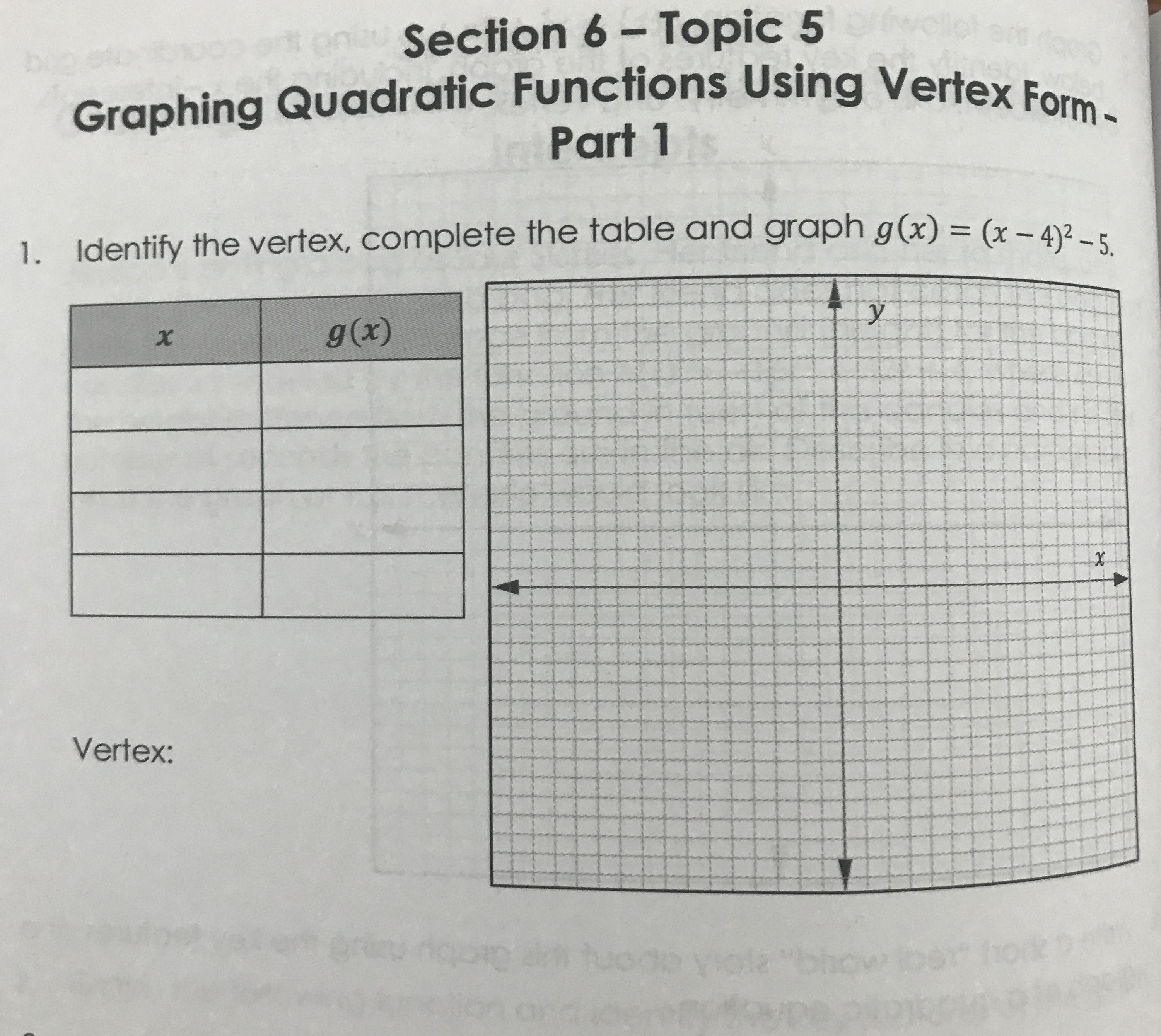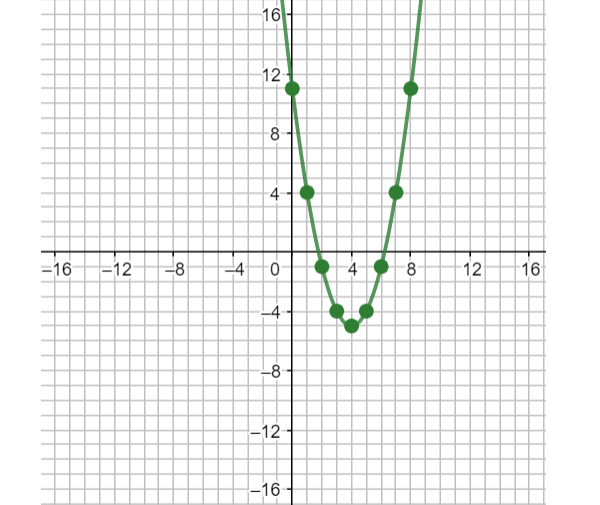# Identify the vertex, complete the table and graph g(x)=(x−4)^2−5.foass77W 2021-02-13 Answered

Identify the vertex, complete the table and graph $g\left(x\right)={\left(x-4\right)}^{2}-5.$You can still ask an expert for help

• Questions are typically answered in as fast as 30 minutes

Solve your problem for the price of one coffee

• Math expert for every subject
• Pay only if we can solve itLayton

The vertex form of a quadratic equation is $y=a{\left(x-h\right)}^{2}+k$ where (h,k) is the vertex.
Comparing $y=a\left(x-h\right)2+k$ and $g\left(x\right)=\left(x-4\right)2-5$ gives h=4 and k=−5. The vertex is then (h,k)=(4,−5).
To complete the table, substitute values of xx on one side of the vertex into g(x) to find the corresponding y-coordinates:
x g(x)
$5\left(5-4\right)2-5=1-5=-4$
$6\left(6-4\right)2-5=4-5=-1$
$7\left(7-4\right)2-5=9-5=4$
$8\left(8-4\right)2-5=16-5=11$
Plot the vertex and the four points from your table. A quadratic is symmetric about its vertex so plot the mirror images of the four points on the other side of the vertex: